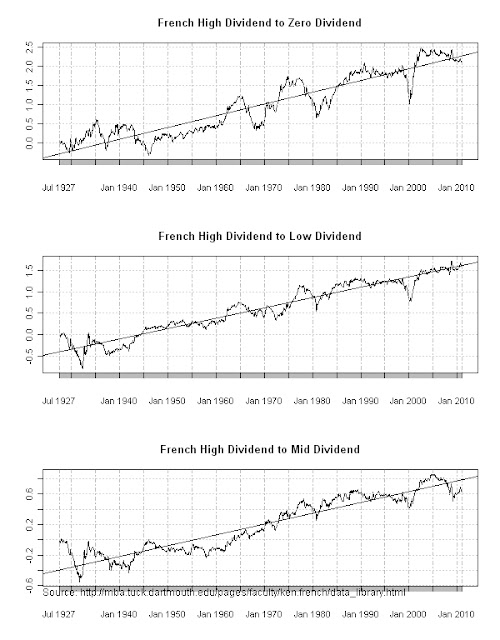Want to share your content on R-bloggers? click here if you have a blog, or here if you don't.

Based on my perception of the last 3 years, I would have expected high dividend stocks to have substantially underperformed low and zero dividend stocks.  Fortunately, just like with size and momentum in Beating Kenneth French Small – High, we can explore the datasets from 1927 with dividend quartiles to see how it looks over the long term.  I thank Kenneth French and R, since I can now disprove my perception.  Strangely the biggest discrepancy from the basic linear model is the high stocks relative to the mid dividend stocks.

For a lot of additional information on high yield stocks, see CSFB Global 2011 Yearbook written by Elroy Dimson, Paul Marsh, and Mike Staunton.From TimelyPortfolioFrom TimelyPortfolioFrom TimelyPortfolio

`#explore dividend data from the#very helpful Ken French#for this project we will look at Dividend Portfolios#http://mba.tuck.dartmouth.edu/pages/faculty/ken.french/ftp/Portfolios_Formed_on_D-P.zip   require(PerformanceAnalytics)require(quantmod)require(ggplot2)   my.url="http://mba.tuck.dartmouth.edu/pages/faculty/ken.french/ftp/Portfolios_Formed_on_D-P.zip"my.tempfile<-paste(tempdir(),"\\frenchmomentum.zip",sep="")my.usefile<-paste(tempdir(),"\\Portfolios_Formed_on_D-P.txt",sep="")download.file(my.url, my.tempfile, method="auto", 	quiet = FALSE, mode = "wb",cacheOK = TRUE)unzip(my.tempfile,exdir=tempdir(),junkpath=TRUE)#read space delimited text file extracted from zipfrench_dividend <- read.table(file=my.usefile,	header = FALSE, sep = "",	as.is = TRUE,	skip = 20, nrows=1002)[,1:5]colnames(french_dividend) <- c("date","Zero","Low","Mid","High")   #get dates ready for xts indexdatestoformat <- french_dividend[,1]datestoformat <- paste(substr(datestoformat,1,4),	substr(datestoformat,5,7),"01",sep="-")   #get xts for analysisfrench_dividend_xts <- as.xts(french_dividend[,2:5],	order.by=as.Date(datestoformat))   french_dividend_xts <- french_dividend_xts/100   #jpeg(filename="performance summary of dividend portfolios.jpg",#	quality=100,width=6.25, height = 8,  units="in",res=96)charts.PerformanceSummary(french_dividend_xts,ylog=TRUE,cex.legend=1.25,	main="Performance by Kenneth French Dividend	Monthly Since 1927",	colorset=c("cadetblue3","cadetblue","cadetblue4",	"darkolivegreen3","darkolivegreen","darkolivegreen4"))mtext("Source: http://mba.tuck.dartmouth.edu/pages/faculty/ken.french/data_library.html",	side=1,adj=0,cex=0.75)#dev.off()   #get price series of small cap high momentum for system building#by applying the cumprod function on each columnfrench_dividend_price <- as.xts(apply(french_dividend_xts[,1:4]+1,FUN="cumprod",MARGIN=2))#if we wanted to compare to the average of all we could use this#french_all_price <- cumprod(1+apply(coredata(french_dividend_xts[,c(1:4)]),MARGIN=1,FUN=mean))   #jpeg(filename="linear models of relative strength dividend.jpg",#	quality=100,width=6.25, height = 8,  units="in",res=96)par(mfrow=c(3,1))for (i in 1:3) {	french_rs <- log(french_dividend_price[,4]/french_dividend_price[,i])	model <- lm(french_rs[,1]~index(french_rs))	plot(french_rs,type="l",		main=paste("French High Dividend to ",colnames(french_dividend_xts)[i]," Dividend",sep=""))	abline(lm(french_rs~index(french_rs)))}mtext("Source: http://mba.tuck.dartmouth.edu/pages/faculty/ken.french/data_library.html",	side=1,adj=0,cex=0.75)#dev.off()   #jpeg(filename="linear residuals of relative strength dividend.jpg",#	quality=100,width=6.25, height = 8,  units="in",res=96)par(mfrow=c(3,1))for (i in 1:3) {	french_rs <- log(french_dividend_price[,4]/french_dividend_price[,i])	model <- lm(french_rs[,1]~index(french_rs))	plot(runSum(as.xts(model\$residuals[,1]),n=24),type="l",		main=paste("Residuals French High Dividend to ",colnames(french_dividend_xts)[i]," Dividend",sep=""))}mtext("Source: http://mba.tuck.dartmouth.edu/pages/faculty/ken.french/data_library.html",	side=1,adj=0,cex=0.75)#dev.off()#another way to chart#	chartSeries(french_rs,theme="white") ,#		TA="addTA(as.xts(model\$fitted.values[,1]),on=1)");addTA(runSum(as.xts(model\$residuals[,1]),n=24))")             ########################################################for later possibly#apply earlier charts and systems from momentum to the dividend data   #speedy solution for ranking from Charles Berry# http://r.789695.n4.nabble.com/efficient-rolling-rank-td2013535.html#rolling rank of price over last 12 months (12 means max price for last 12)#pad first 11 with NAnper <- 10x.rank <- c(rep(NA,nper-1),rowSums(coredata(french_high_price)[ -(1:(nper-1)) ] >= embed(french_high_price,nper)))x.rank <- as.xts(x.rank,order.by=index(french_high_price))signalRank <- ifelse(x.rank[,1] > 3 | 	french_high_price >= runMax(french_high_price,2),1,0)retRank <- lag(signalRank,k=1)*french_dividend_xts[,4]   #try rolling 10 month moving average popularized by Mebane FabersignalAvg <- ifelse(french_high_price > runMean(french_high_price,n=10),1,0)retAvg <- lag(signalAvg,k=1)*french_dividend_xts[,4]   #try RSIsignalRSI <- ifelse(RSI(french_high_price,n=4) > 45,1,0)retRSI <- lag(signalRSI,k=1)*french_dividend_xts[,4]   retCompare <- merge(retRank,retAvg,retRSI,french_dividend_xts[,4])colnames(retCompare) <- c("Small.High.Rank",	"Small.High.Avg","Small.High.RSI","Small.High.Benchmark")#jpeg(filename="performance of small-high with systems.jpg",quality=100,width=6.25, height = 8,  units="in",res=96)charts.PerformanceSummary(retCompare,ylog=TRUE,cex.legend=1.2,	colorset = c("cadetblue","darkolivegreen3","purple","gray70"),	main="Kenneth French Small Size and High Momentum Stocks	Compared To Various Price Systems")#dev.off()   #jpeg(filename="capture of small-high with systems.jpg",quality=100,width=6.25, height = 8,  units="in",res=96)chart.CaptureRatios(retCompare[,1:3],retCompare[,4],	main="Kenneth French Small Size and High Momentum Stocks	Compared To Various Price Systems")#dev.off()   #get average of small size for additional system testingfrench_dividend_avg <- cumprod(1+apply(coredata(french_dividend_xts[,c(1:4)]),MARGIN=1,FUN=mean))french_dividend_avg <- as.xts(french_dividend_avg,order.by=index(french_high_price))nper <- 10x.rank <- c(rep(NA,nper-1),rowSums(coredata(french_dividend_avg)[ -(1:(nper-1)) ] >= embed(french_dividend_avg,nper)))x.rank <- as.xts(x.rank,order.by=index(french_dividend_avg))signalRankAvg <- ifelse(x.rank[,1] > 3 | 	french_dividend_avg >= runMax(french_dividend_avg,2),1,0)retRankAvg <- lag(signalRankAvg,k=1)*french_dividend_xts[,4]   retCompare <- merge(retRank,retRankAvg,french_dividend_xts[,3])colnames(retCompare) <- c("Small.High.Rank",	"Small.High.RankOnAvg","Small.High.Benchmark")#jpeg(filename="performance of small-high with 2 rank systems.jpg",quality=100,width=6.25, height = 8,  units="in",res=96)charts.PerformanceSummary(retCompare,ylog=TRUE,cex.legend=1.2,	colorset = c("cadetblue","darkolivegreen3","gray70"),	main="Kenneth French Small Size and High Momentum Stocks	Compared To Rank-based Price Systems")#dev.off()       chart.QQPlot(french_dividend_xts[,4],	 main = "Normal Distribution", distribution = 'norm', envelope=0.95)library(MASS)fit = fitdistr(1+french_dividend_xts[,3], 'lognormal')chart.QQPlot(1+french_dividend_xts[,3], main = "Log-Normal Distribution", envelope=0.95, distribution='lnorm', meanlog = fit\$estimate[], sdlog = fit\$estimate[])library(sn)fit = st.mle(y=french_dividend_xts[,3])chart.QQPlot(french_dividend_xts[,3], main = "Skew T Distribution", envelope=0.95, distribution = 'st', location = fit\$dp[], scale = fit\$dp[], shape = fit\$dp[], df=fit\$dp[])       chart.Histogram(french_dividend_xts[,4], methods = c( "add.density", "add.normal") )chart.Histogram(french_dividend_xts[,1], methods = c( "add.centered", "add.density", "add.rug") )chart.Histogram(french_dividend_xts[,3], methods = c( "add.centered", "add.density", "add.rug", "add.qqplot") )chart.Histogram(french_dividend_xts[,3], methods = c("add.density", "add.centered", "add.rug", "add.risk") )     #interesting linearity between momentumchart.Regression(french_dividend_xts[,4], apply(coredata(french_dividend_xts[,c(1:4)]),MARGIN=1,FUN=mean),	 fit="conditional", main="Conditional Beta")`